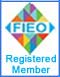Note: No part of this website may be reproduced, stored in a retrieval, or transmitted in any form or by any mean

## Our ProductsHome » Physics Laboratory Instruments   »   Stefan's Constant Kit Stefan's Constant Kit OBJECTIVE: Sefan's constant by the black copper radiation plates (Electrical Method).Stefan's Constant Kit Code: SCK-001 Stefan's Constant KitAPPARATUS: The KIT contains two black copper radiation plates with heater element in between, Thermocouple, built in power supply, a.c. Voltmeter, a.c. Ammeter and their controls. The KIT is self-contained and needs no additional equipment. THEORY: The electricity fed to the heater element is radiated by radiating disc whose steady state temperature gives the total power radiated. If E is the total amount of heat radiated per unit area of the body per unit time and T is the absolute temperature of the body, then according to Stefan's law:Whereis the Stefan's constant. If the body is put in a surrounding of temperature then it is also receiving an amount of heat radiation given byThe net amount of heat radiates given out by the body per unit area per unit time is therefore.If A is the total area of the body given out the heat radiation then the net heat radiated per unit time is given byIn the present case if we neglect the heat transfer on account of conduction and convention of the air in contact, heat energy radiated by copper discs per unit time is equal to the power supplied to heater element. Therefore if V is the potential difference across the heater and I is the current passing through it, then,From which the Stefan's constant is determined V, I & T are measured from Voltmeter, Ammeter and Temperature Indicator provided in the KIT. SALIENT FEATURES There is practically no loss of heat energy from heating element by conduction, convection or radiation Therefore, entire heat energy of the heating element is transferred to the discs. As the efficiency of the heating element is nearly equal to one, almost entire electrical energy supplied to the heater will be converted in heat energy